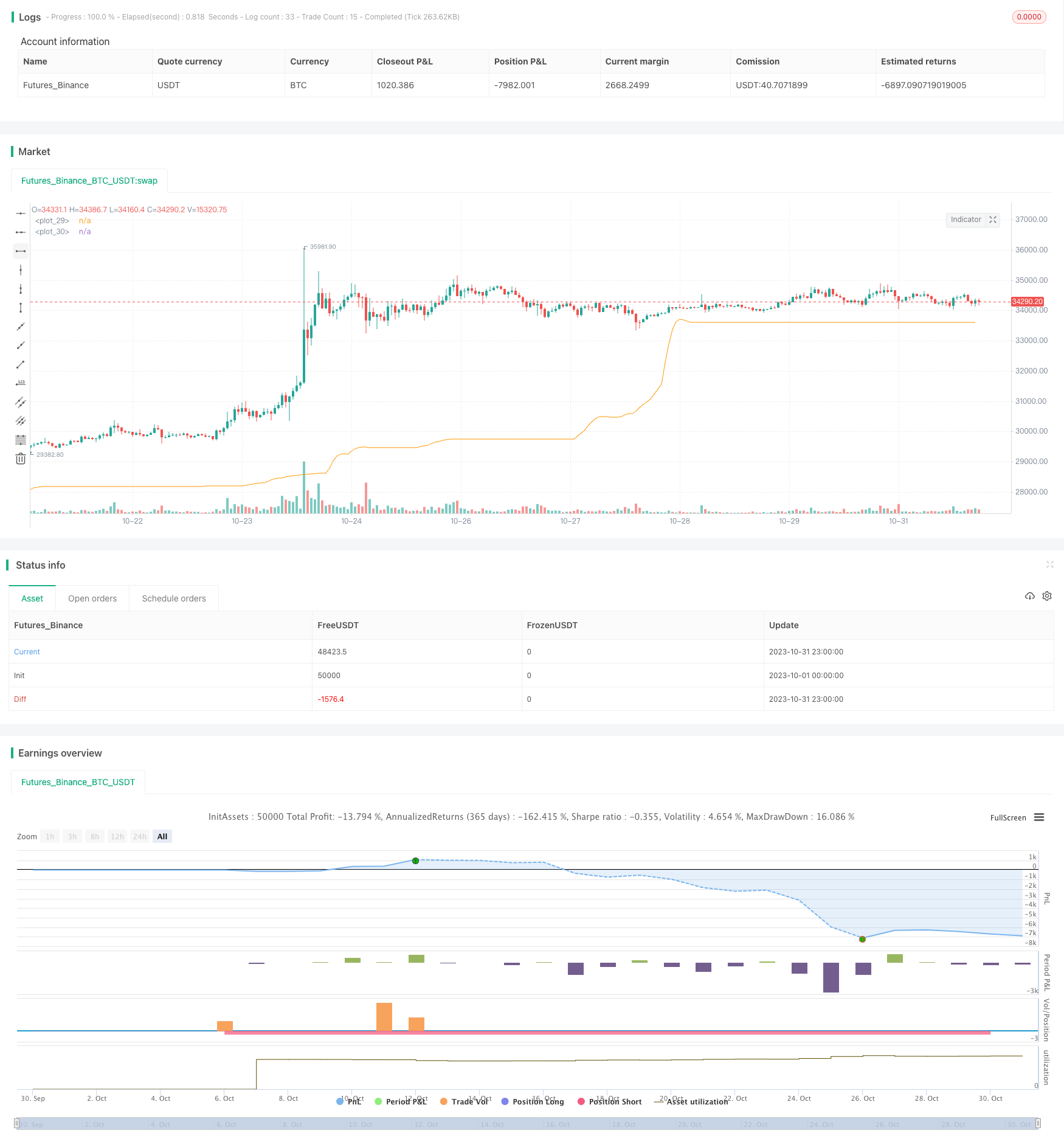# Adaptive Regularized Moving Average Cross-Market Arbitrage Strategy

Author: ChaoZhang, Date: 2023-11-16 16:20:11
Tags:## Overview

This strategy implements arbitrage trading between different markets by calculating an adaptive regularized moving average line. The strategy features cross-market arbitrage, dynamic parameter adjustment, risk control, etc.

## Strategy Principle

The strategy first defines a scaleMinimax function to standardize the time series to a specified range. Then it defines an adaptive regularized moving average function rema to calculate the smoothed signal line sig. The calculation of the signal line is:

1. Define a sliding window, default length 5 days.

2. The sig value for each day is the weighted average of the previous sig value and the current closing price. The weighting uses an adaptive weighting mechanism, where values closer to the current price are weighted higher.

3. Add a λ parameter as a regularizer to make the sig transition smoother.

After obtaining the signal line, the strategy determines long/short based on the golden/dead cross of the signal line and price. Specifically:

1. When sig crosses above price, go long.

2. When sig crosses below price, go short.

Compared with traditional moving average strategies, this strategy has the following advantages:

1. The adaptive weighting mechanism can respond faster to price changes.

2. The added regularizer makes the signal line smoother, avoiding wrong signals from drastic price fluctuations.

3. Cross-market arbitrage can profit from price differences between markets.

4. Flexible adjustable parameters can be optimized according to market conditions.

## Risks and Solutions

The strategy also has some risks:

1. The probability of wrong signals from double crossovers is higher. The solution is to properly adjust the smooth parameter to avoid oscillation of the signal line.

2. Cross-market arbitrage requires that the two markets have price correlation and consistent trends. The solution is to select highly correlated markets for arbitrage.

3. Parameter optimization requires sufficient historical data for backtesting. The solution is to cautiously adjust parameters in live trading.

## Optimization Directions

The strategy can also be optimized in the following aspects:

1. In parameter selection, machine learning algorithms can be introduced to automatically optimize parameter combinations.

2. In signal generation, more indicators can be introduced to construct more stable trading signals.

3. In risk control, stop loss can be set to limit the loss per trade.

4. In cross-market arbitrage, it can be extended to more highly correlated trading assets.

## Summary

This strategy implements arbitrage trading between markets by adaptively calculating moving averages. Compared with traditional moving average strategies, it has the advantages of adaptive parameters, smoothed processing, cross-market arbitrage, etc. Next steps are to further optimize the strategy through machine learning, combined signals, risk management, etc.

```/*backtest
start: 2023-10-01 00:00:00
end: 2023-10-31 23:59:59
period: 1h
basePeriod: 15m
exchanges: [{"eid":"Futures_Binance","currency":"BTC_USDT"}]
*/

//@version=3
strategy("Crossover82%", overlay=true)

//
// Functions
//
scaleMinimax(X, p, min, max) =>
hi = highest(X, p), lo = lowest(X, p)
(max - min) * (X - lo)/(hi - lo) + min

rema(ts, p) => // regularized ma
rm = 0.0, lambda = .5, a = 2 / (p + 1)
rm := (nz(rm) + a * (ts - nz(rm)) + lambda * (2 * nz(rm) - nz(rm))) / (lambda + 1)
rm

//
// Inputs
//
X = input(close, title="Data source")
smooth = input(2, title="REMA smooth factor")
show_line = input(true, title="Show signal line")

//
// Main
//
p = 5
sig = rema(scaleMinimax(pow(X*p,-X) - 0.1, 100, lowest(X, 100), highest(X, 100)), smooth)

plot(show_line ? sig : na, linewidth=1)
plot(cross(sig, X) ? ohlc4 : na, style=circles, linewidth=8, color=blue, transp=50)

longCondition = crossover(sig, X)
if (longCondition)
strategy.entry("LE", strategy.long)

shortCondition = crossunder(sig, X)
if (shortCondition)
strategy.entry("SE", strategy.short)

```

More